# Central limit theorem facts for kids

Kids Encyclopedia Facts

In probability theory and statistics, the central limit theorems, abbreviated as CLT, are theorems about the limiting behaviors of aggregated probability distributions. They say that given a large number of independent random variables, their sum will follow a stable distribution. If the variance of the random variables is finite, then a Gaussian distribution will result. This is one of the reasons why this distribution is also known as normal distribution.

The best known and most important of these is known as the central limit theorem. It is about large numbers of random variables with the same distribution, each with an identical finite variance and expected value.

More specifically, if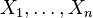$X_1, \ldots, X_n$ are n identical and independently distributed random variables with mean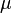$\mu$ and standard deviation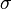$\sigma$, then the distribution of their sample mean,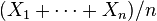$(X_1 + \cdots + X_n)/n$, as n gets large, is approximately normal with mean$\mu$ and standard deviation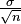$\tfrac{\sigma}{\sqrt{n}}$. Furthermore, the distribution of their sum,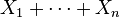$X_1 + \cdots + X_n$, as n gets large, is also approximately normal, with mean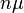$n\mu$ and standard deviation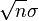$\sqrt{n}\sigma$.

There are different generalisations of this theorem. Some of these generalisations no longer require an identical distribution of all random variables. In these generalisations, another precondition makes sure that no single random variable has a bigger influence on the outcome than the others. Examples are the Lindeberg and Lyapunov conditions.

The name of the theorem is based on a paper George Pólya written in 1920, About the Central Limit Theorem in Probability Theory and the Moment problem.

## Images for kidsCentral limit theorem Facts for Kids. Kiddle Encyclopedia.